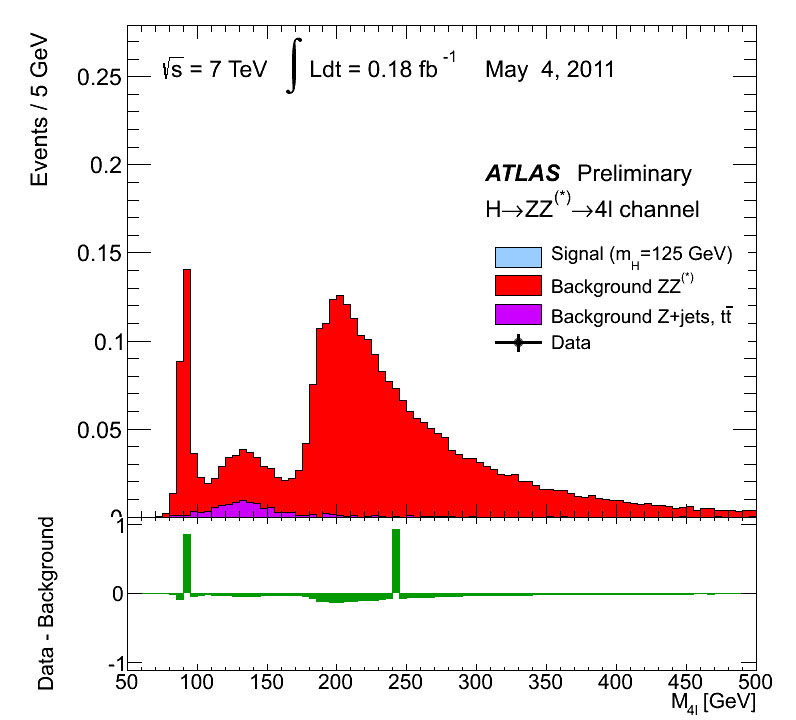The data for Higgs bosons come with a background

The red and purple parts of the histogram represent the "background," which is the number of 4-lepton events we predict will occur from decays not involving a Higgs boson.

The blue part (following page) represents the "signal" prediction, which is the number of 4-lepton events we predict will result from Higgs boson decays. The signal is stacked on top of the background, because to get the total predicted number of events, you simply add up all the possible ways the event can occur.

The black dots represent the number actually observed, while the black lines through the dots represent the statistical uncertainty in those numbers. The bump in the data (following page) at around 125 GeV is one sign for the new 125 GeV particle (the Higgs boson).Mass plot showing backgrounds for the decay Higgs boson → two Z bosons → four leptons. These backgrounds are the direct production of two Z bosons without any Higgs involved, or from rare decays of a single Z boson to four leptons, or other such backgrounds. The Higgs boson to two Z bosons followed by their decay to four leptons has to be seen on top of these backgrounds. © CERN